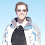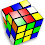## Friday, February 22, 2008

### Use the digits 1 through 9 exactly once...

This is a quick puzzle that shouldn't be too difficult to figure out.

Q: Arrange the digits 1 through 9 to form three 3-digit perfect squares. You must use each of the nine digits exactly once.

1.The squares of the numbers between 10 and 31 are the only 3-digit ones, and eliminating those with repeated digits leaves only 13 choices (of which 169, 196, and 961 are equivalent).
A little playing around led to
361, 529, 784.

2.I had a tough time understanding what the clue meant. Now that I have seen Eric's response, it makes perfect sense. I didn't have the time to ask, but will endeavor to do so next time. Thanks, this was a clever one!

3.Compute 10² through 31². That will give you all the 3-digit perfect squares. Now eliminate any with repeated digits within them. Also, several of them use the same set of digits, so I’ve grouped them together. That leaves 10 groups of squares.
169, 196, 961
256, 625
289
324
361
529
576
729
784
841

There are only two that contain the digit 3 (324 and 361), so let’s work with them.

If you pick 324, you can eliminate squares with those digits: That leaves:
169, 196, 961
576
But there is no square with an 8 left, so this won’t.

We know that 361 will be one of the squares. You can eliminate squares with those digits: That leaves:
289
529
729
784

You need a 4, so 784 will be one of the squares.
You need a 5, so 529 will be one of the squares.

Checking you see that all the digits appear exactly once in these 3 squares: